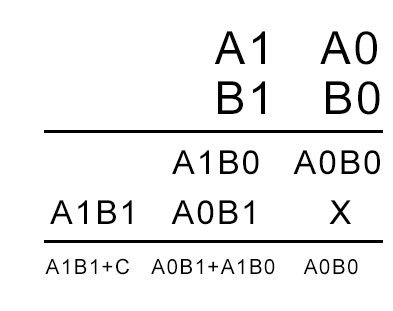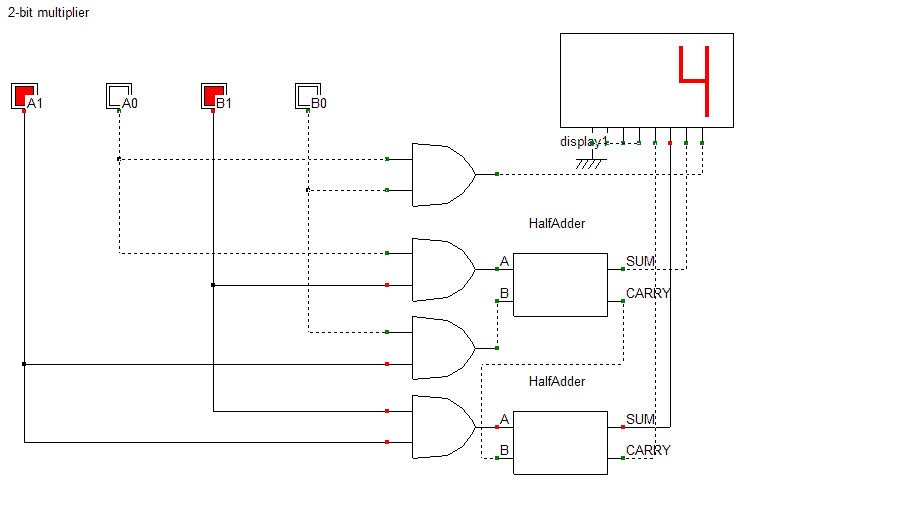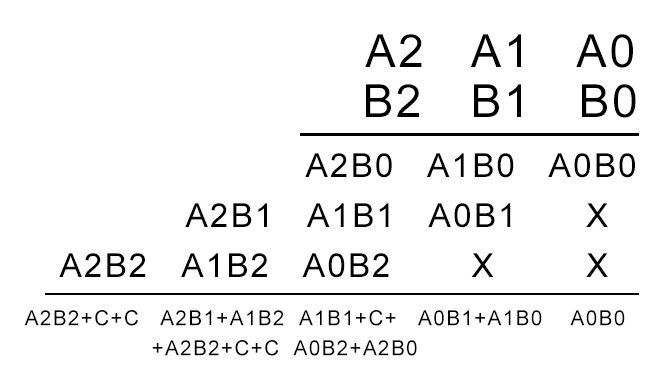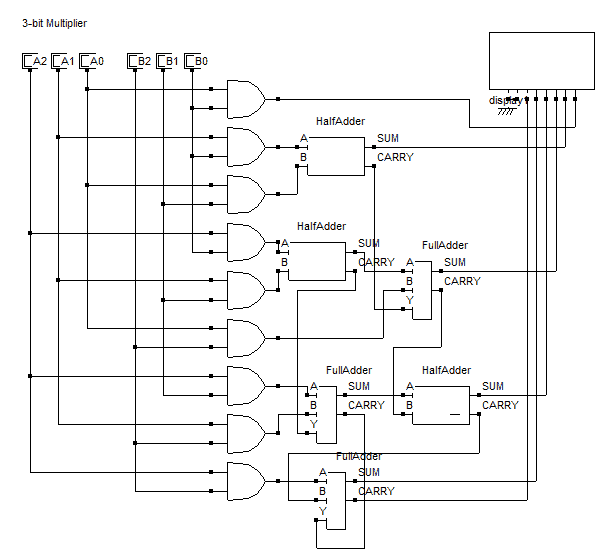Multiplier – Designing of 2-bit and 3-bit binary multiplier circuits

A multiplier is a combinational logic circuit that is used to multiply binary digits. Just like the adder and the subtractor, a multiplier is an arithmetic combinational logic circuit. It is also known as a binary multiplier or a digital multiplier.

Where is the use of a multiplier?

It is used in several digital signal processing applications. It is used to design calculators, mobiles, processors and digital image processors.

How does binary multiplication work and how to design a 2-bit multiplier?

It works just like normal multiplication. There are four main rules that are quite simple to understand:

0  x  0  =  0

0  x  1  =  0

1  x  0  =  0

1  x  1  =  1

Suppose you have two binary digits A1A0 and B1B0, here’s how that multiplication would take placeIn the above calculation, A1A0 is the multiplicand. B1B0 is the multiplier. The first product obtained from multiplying B0 with the multiplicand is called as partial product 1. And the second product obtained from multiplying B1 with the multiplicand is known as the partial product 2. As the number of bits increases, we keep shifting each successive partial product to the left by 1 bit. In the end, we add the digits while keeping in mind the carry that might generate.

Based on the above equation, we can see that we need four AND gates and two half adders to design the combinational circuit for the multiplier. The AND gates will perform the multiplication and the half adders will add the partial product terms. Hence the circuit obtained is as follows.How to design a 3-bit multiplier?

Consider two general 3-bit binary numbers A2A1A0 and B2B1B0. Multiplying the two number with each other using standard binary multiplication rules, we get the following equation.Adding A2B0 and A1B1 will give rise to one carry, adding the sum obtained from that and the carry obtained from adding A1B0 and A0B1 to A0B2 will give rise to another carry. Thus, two carries are generated and are carried over to the addition between A2B1 and A1B2, where two more carries are generated in a similar fashion. Hence the resulting circuit will contain nine AND gates, three half adders and three full adders. The resultant circuit is given below.Note: We will use all of the equations above when we code multipliers using VHDL in our VLSI course

This site uses Akismet to reduce spam. Learn how your comment data is processed.